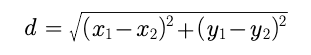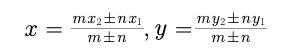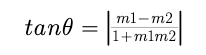# SHORT FORMULA - MATHEMATICS - STRAIGHT LINE

SHORT FORMULA - MATHEMATICS - STRAIGHT LINE

Distance Formula :Section Formula :Angle between two straight lines :Post By : Rahul Kumar 10 Apr, 2020 2441 views Maths